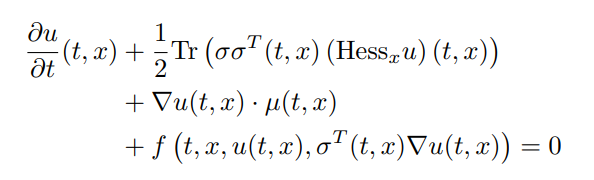# Deep Forward-Backwards SDEs for Terminal Parabolic PDEs

To solve high-dimensional PDEs, one should first describe the PDE in terms of the TerminalPDEProblem with constructor:

TerminalPDEProblem(g,f,μ_f,σ_f,X0,tspan,p=nothing)

which describes the semilinear parabolic PDE of the form:with terminating condition u(tspan,x) = g(x). These methods solve the PDE in reverse, satisfying the terminal equation and giving a point estimate at u(tspan,X0). The dimensionality of the PDE is determined by the choice of X0, which is the initial stochastic state.

To solve this PDE problem, there exist two algorithms:

• NNPDENS(u0,σᵀ∇u;opt=Flux.ADAM(0.1)): Uses a neural stochastic differential equation, which is then solved by the methods available in DifferentialEquations.jl. The alg keyword is required for specifying the SDE solver algorithm that will be used on the internal SDE. All of the other keyword arguments are passed to the SDE solver.
• NNPDEHan(u0,σᵀ∇u;opt=Flux.ADAM(0.1)): Uses the stochastic RNN algorithm from Han. Only applicable when μ_f and σ_f result in a non-stiff SDE where low order non-adaptive time stepping is applicable.

Here, u0 is a Flux.jl chain with a d-dimensional input and a 1-dimensional output. For NNPDEHan, σᵀ∇u is an array of M chains with a d-dimensional input and a d-dimensional output, where M is the total number of timesteps. For NNPDENS it is a d+1-dimensional input (where the final value is time) and a d-dimensional output. opt is a Flux.jl optimizer.

Each of these methods has a special keyword argument pabstol, which specifies an absolute tolerance on the PDE's solution, and will exit early if the loss reaches this value. Its default value is 1f-6.